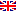Scheda programma d'esame
ISTITUZIONI DI ANALISI NUMERICA
DARIO ANDREA BINI
CdSMATEMATICA
Codice136AA
CFU9
PeriodoSecondo semestre
LinguaItaliano

ModuliSettore/iTipoOreDocente/i
ISTITUZIONI DI ANALISI NUMERICAMAT/08LEZIONI63
 DARIO ANDREA BINI unimap BEATRICE MEINI unimapEsporta in pdf
Learning outcomes
Knowledge

Students will learn and practice the theoretical and computational aspects in the area of approximation theory, orthogonal polynomials, numerical integration, and partial differential equations.

Assessment criteria of knowledge

The student will be assessed on his/her demonstrated ability

• tto discuss the main course contents using the appropriate terminology
• to solve exercises
• to relate and compare different topics and techniques encountered in the course
• to report properties, theorems and their proofs

Methods:

• Final oral exam
• Final written exam
Skills

The student who successfully completes the course will have the ability to look at problems in approximation theory and in differential equations from the computational point of view. He/she will have the basic tools and the capabilities to learn more advanced tools and and algorithmic solutions. He/she will have the basis concepts needed for performing the design and analysis of algorithms and for approaching research topics.

Assessment criteria of skills

The assessment criteria of skills rely on solving suitable exercises concerning different parts of the course.

Behaviors

Students will be able to read and analyze research results, design and analyze algorithms for solving numerical problems

Assessment criteria of behaviors

Solving suitable and nonstandard exercises concerning different parts of the course are once again the main criteria for the assessment of behaviors.

Prerequisites

Basic notions of linear algebra, functional analysis and numerical analysis.

Teaching methods

Learning activities:

• attending lectures
• individual study

Teaching methods:

• frontal Lectures
Syllabus

1- Orthogonal polynomials: properties, interplay with tridiagonal matrices; specific polynomials: Gegenbauer, Chebyshev, Legendre, Hermite polynomials;

2- Numerical integration. Newton-Cotes,, Clenshaw-Curtis and Gaussian formulae.

3- Approximation of continuous function. Best approximation in Banach and in Hilbert spaces. Computational aspects. Minimax approximation.  Spline functions. Rational approximation. Matrix functions.

4- Numerical treatment of partial differential equations by means of finite differences methods: The Poisson problem, the heat equation, the wave equation.

Bibliography

Useful readings include the following works

• R. Bevilacqua, D.A. Bini, M. Capovani, O. Menchi, Metodi Numerici, Zanichelli, 1992
• D.A. Bini, M. Capovani, O. Menchi, "Metodi numerici per l'algebra lineare", Zanichelli, 1988.
• Eugene Isaacson and Herbert Bishop Keller, Analysis of Numerical Methods. Jhon Wiley & Sons, Inc., New York, 1966.
• R.J. LeVeque. Finite Differences Methods for Ordinary and Partial Differential Equations. SIAM 2007.
• W. Rudin, Real and Complex Analysis, Second Edition, Tata McGraw-Hill, 1974.
• J. Stoer, R. Burlisch, Introduction to Numerical Analysis, Third Edition, Springer, 2002.
• Lectures notes supplied by the lecturer
Assessment methods
• Final written exam
• Final oral exam
Pagina web del corso

https://elearning.dm.unipi.it

Ultimo aggiornamento 27/07/2017 15:26